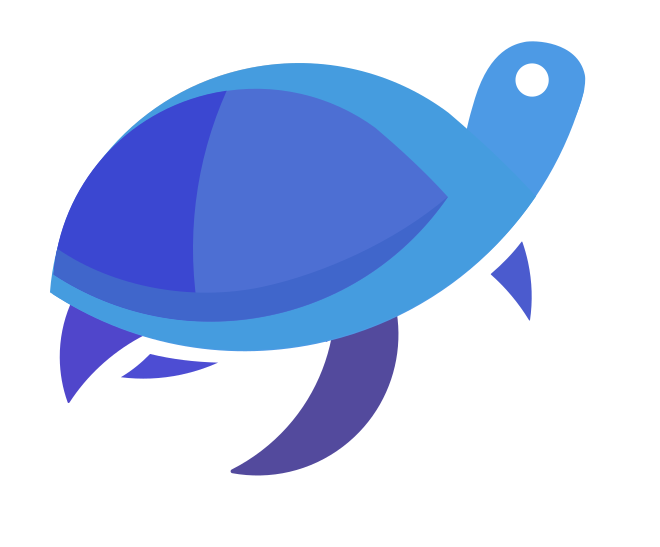Learn
Turtle
Way to Matplotlib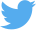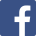Learn
Turtle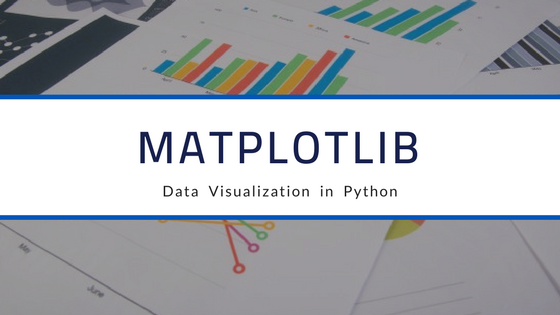Way to MatplotlibWay to Matplotlib

## What is Matplotlib?

Matplotlib is an open-source ,plotting library in python for 2D plots of arrays.It is a multi-platform data visualization library built on NumPy arrays and designed to work with the broader SciPy stack.It was originally devised by John Hunter in the year 2002.## Features -

Matplotlib has a great ability to play with many operating systems and graphics backends. It supports various backends and output types. Moreover, being a cross platform, it has led to a multitude of contributors and user base, which ultimately has led to an active developer base and Matplotlib’s powerful tools and universalness within the scientific Python environment.

## Installation-

Windows, Linux and macOS distributions have most of matplotlib dependencies as wheel packages. To install matplotlib packages run the following command :

``````python -m pip install -U pip
python -m pip install -U matplotlib

``````

Using Anaconda, you can simply install Matplotlib from your terminal or command prompt using:

`````` conda install matplotlib

``````

## Importing Matplotlib-

we will use plt shorthand for Matplotlib imports:

`````` import matplotlib.pyplot as plt
#or
from matplotlib import pyplot as plt

``````

## Some types of plots represented using Matplotlib

Python Matplotlib comes with a wide variety of plots. Plots helps to understand trends, patterns, and to make correlations in easier way. Some of them are listed below:

1. Line plot -

A line chart or line graph is a type of chart which displays information as a series of data points called ‘markers’ connected by straight line segments. It is one of the most basic type of chart and it is common in many fields. It is more or less similar to a scatter plot except that the measurement points are ordered (typically by their x-axis value) and joined with straight line segments.2. Histogram -

It is an accurate and easy representation of the distribution of numerical data. It is an estimate of the probability distribution of a continuous variable. The hist() func will generate histograms and then return the bin counts or probabilities.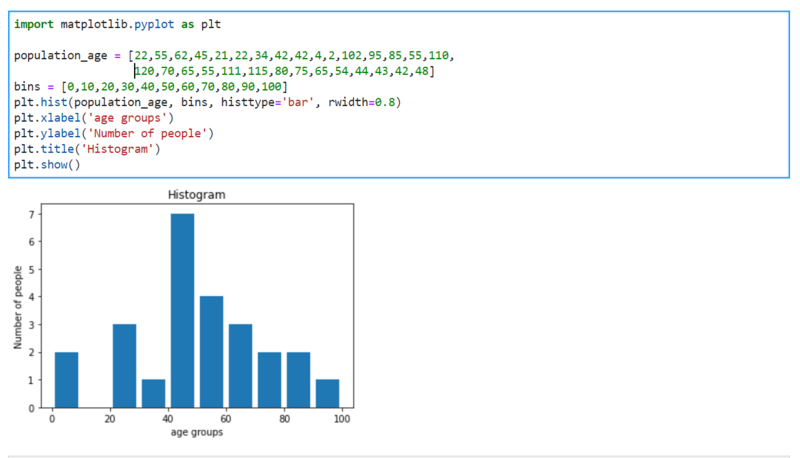3. Scatter plot –

Scatter plot is a classic and fundamental plot used to study the relationship between two variables. Sometime we have multiple groups in our data so we want to visualize each group in different color. In matplotlib, you can do this using plt.scatterplot().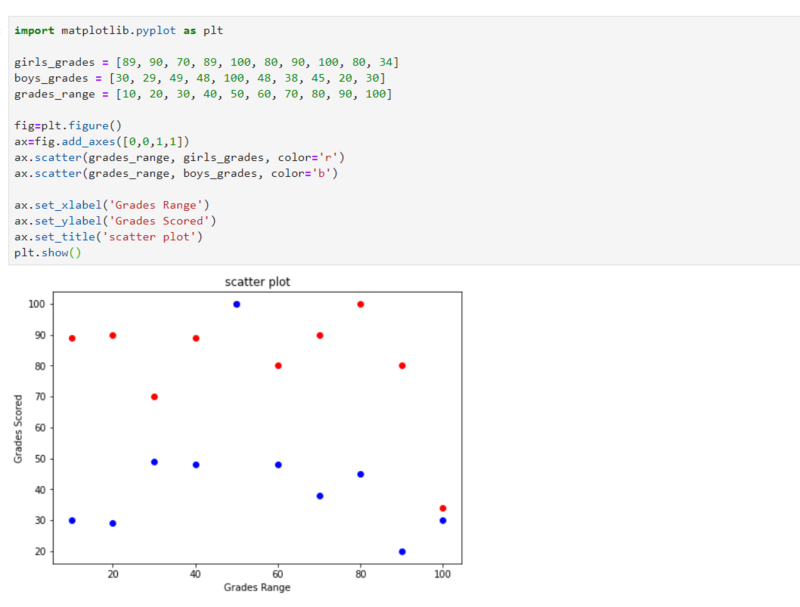4. Pie plot -

It is a classic way to show the composition of groups. A Pie Chart can only display one series of data. Pie charts show the size of items (called wedge) in one data series, proportional to the sum of the items. The data points in a pie chart are shown as a percentage of the whole pie.So, if you are to use pie chart, its highly recommended to explicitly write down the percentage or numbers for each portion of the pie.5. Bar graph -

A bar graph uses bars to 8compare data among different categories. It is well suited when you want to measure the changes over a period of time. It can be represented horizontally or vertically.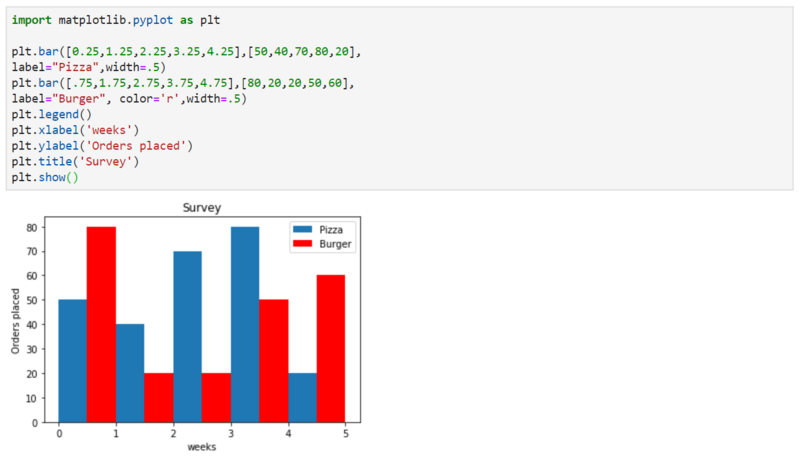6. Contour plots -

(sometimes called Level Plots) are a way to show a 3-D surface on a 2-D plane. Contour graphs uses two predictor variables X, Y on the y-axis and a response variable Z as contours. These contours are called the z-slices or the iso-response values.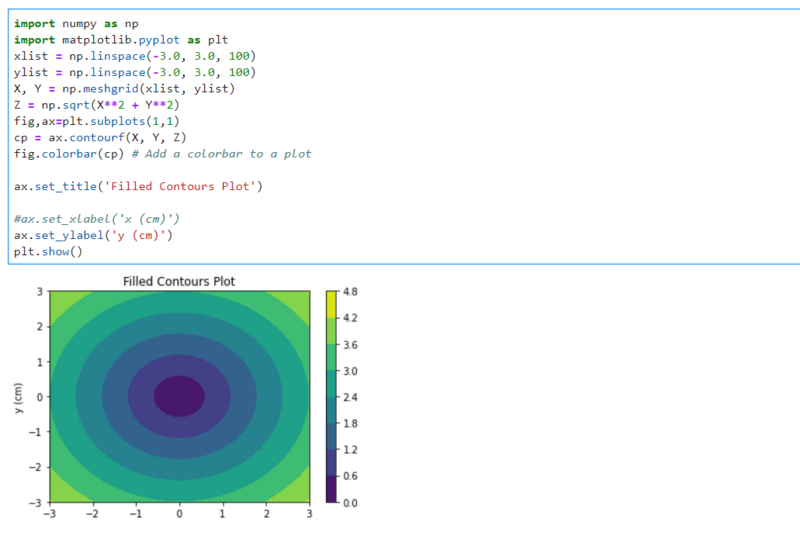7. Violin plots -

Violin plots are similar to box plots, except that they also show the probability density of the data at different values. These plots include a marker for the median of the data and a box indicating the interquartile range, as in the standard box plots. Overlaid on this box plot is a kernel density estimation.They show the probability density of the data at different values.This is just an introduction to matplotlib in which I've tried to show you basics of making visualizations using it and how you can approach visualization of some important plots.For more learning resources,real python and Matplotlib documentation are a great places to look. Let me know if you find this article useful or wants to know more about it. If this article was helpful to you in some way, show me some support in comment section.